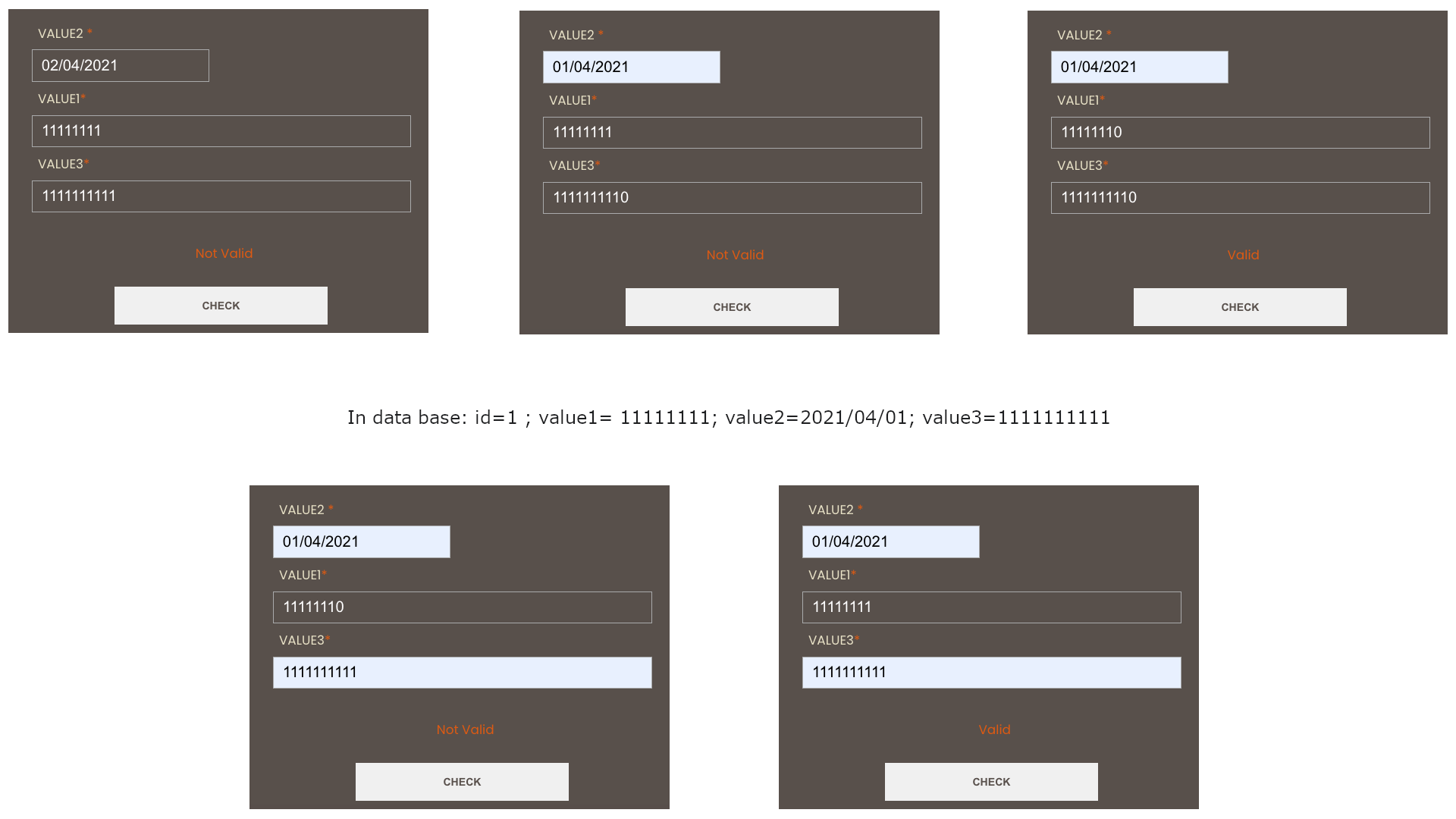# Check combination of values in Laravel

I have three columns (input1, input2, input3), I need to compare them and check that there is not a combination of the values already registered in the database.

I need to return `true` when they are all the same or when they are all different. In intermediate cases, return `false`.

I tried:

```public function check(\$input1, \$input2, \$input3) {
if (Model::where('input1', 'LIKE', \$input1)->where('input2', 'LIKE', \$input2)->where('input3', '=', \$input3)->exists()) {
return false;
} else {
if (Model::where('input1', '=', \$input1)->where('input3', '!=', \$input3)->exists()) {
return true;
}

if (Model::where('input1', '!=', \$input1)->where('input3', '=', \$input3)->exists()) {
return true;
}

if (Model::where('input1', '=', \$input1)->where('input3', '=', \$input3)->exists()) {
return true;
}
}
}```

Not being met when `input1` and `input3` are registered, but no `input2` is found in the table.

How can I solve it, the idea is to compare the three inputs.

Thanks!

UPDATE:

I tried:

```public function check(\$input1, \$input2, \$input3) {
if (Model::where('input1', '=', \$input1)->where('input3', '!=', \$input3)->exists()) {
return true;
}
if (Model::where('input1', '!=', \$input1)->where('input3', '=', \$input3)->exists()) {
return true;
}
if (Model::where('input1', '=', \$input1)->where('input3', '=', \$input3)->exists()) {
return true;
}
if (Model::where('input1', '!=', \$input1)->where('input3', '!=', \$input3)->exists()) {
return false;
}
}```

I need to correct the above so that I can validate when all three values match a record.

UPDATE:

I need to validate according to the imageUPDATE:

is an example of what i need to doUPDATE:

A resolution strategy could be, to verify only the cases when the three values coincide completely or when they are totally different

```public function check(\$input1, \$input2, \$input3)
{
// initialization
\$temp1=true;
\$temp1=true;

if (Model::where('input1', '=' ,\$input1)->whereDate('input2', '=' ,\$input2)->where('input3', '=' ,\$input3)->exists()){
return true;
} else {
\$temp1=false;
}

if (\$temp1=false && Model::where('input1', '!=' ,\$input1)->whereDate('input2', '!=' ,\$input2)->where('input3', '!=' ,\$input3)->exists()){
return true;
} else {
\$temp2=false;
}

if (\$temp1=false && \$temp2=false){
return false;
}
}```
Contents

### Method 1

Okay, you can do it in one simple query, what you want (logically speaking) is an SQL query like:

``````SELECT *
FROM table
WHERE (
(value1 = ?) OR
(value2 = ?) OR
(value3 = ?)
)
``````

If this returns 1 row or more, then you want to return `false`. So, you can take advantage of `exists` method. It will use `EXISTS` SQL method or whatever is called.

So, you can do 2 different things, the first one I am going to show is the same you are doing, but I don’t know if you are using this in a controller, so you could use it in a `FormRequest` or `validator`.

``````public function check(\$value1, \$value2, \$value3)
{
return !Model::where('value1', \$value1)->where('value2', \$value2)->where('value3', \$value3)->exists();
}
``````

See that I wrote `!` before `Clientes`, so if these data exists, `exists()` will return `true`, but you want to opposite in this case.

#### Another way

Now, if you want to use it in a `validator` or a `FormRequest`, where you write the rules, you should have something like this:

``````use IlluminateSupportFacadesValidator;

Validator::validate(
[
'value1' => \$value1,
'value2' => \$value2,
'value3' => \$value3,
],
[
'value1' => 'unique:clientes',
'value2' => 'unique:clientes',
'value3' => 'unique:clientes',
]
);
``````

Take in mind that, to do the inverse of `exists`, we use `unique`. If any of the values exist, then it will throw an error and return to the view (but this should only be used in `Controller`s or `FormRequest`s (rules, not `validator` literally)).

Update:

To have a better code, do this:

``````public function check(\$input1, \$input2, \$input3)
{
if (Model::where('input1', \$input1)->whereDate('input2', \$input2)->where('input3', \$input3)->exists()) {
return true;
} else if (Model::where('input1', '!=', \$input1)->whereDate('input2', '!=', \$input2)->where('input3', '!=', \$input3)->exists()) {
return true;
}

return false;
}
``````

Still, this query could get better just doing one into two, but I am not 100% sure how to write that now.

And still makes not much sense (at least to me) to check if it exactly matches a row (at least with some fields), or totally the opposite, don’t match any row with those values. It is a XOR gate but makes no sense.